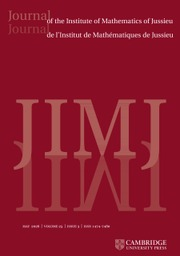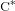Home
Hostname: page-component-59b7f5684b-569ts Total loading time: 0.87 Render date: 2022-10-04T08:43:01.256Z Has data issue: true Feature Flags: { "shouldUseShareProductTool": true, "shouldUseHypothesis": true, "isUnsiloEnabled": true, "useRatesEcommerce": false, "displayNetworkTab": true, "displayNetworkMapGraph": true, "useSa": true } hasContentIssue trueJournal of the Institute of Mathematics of Jussieu

## Abstract

HTML view is not available for this content. However, as you have access to this content, a full PDF is available via the ‘Save PDF’ action button.

We completely classify Cartan subalgebras of dimension drop algebras with coprime parameters. More generally, we classify Cartan subalgebras of arbitrary stabilised dimension drop algebras that are non-degenerate in the sense that the dimensions of their fibres in the endpoints are maximal. Conjugacy classes by an automorphism are parametrised by certain congruence classes of matrices over the natural numbers with prescribed row and column sums. In particular, each dimension drop algebra admits only finitely many non-degenerate Cartan subalgebras up to conjugacy. As a consequence of this parametrisation, we can provide examples of subhomogeneous$\text{C}^{\ast }$-algebras with exactly$n$ Cartan subalgebras up to conjugacy. Moreover, we show that in many dimension drop algebras two Cartan subalgebras are conjugate if and only if their spectra are homeomorphic.

## MSC classification

Type
Research Article
Information
Creative CommonsThis is an Open Access article, distributed under the terms of the Creative Commons Attribution licence (http://creativecommons.org/licenses/by/4.0/), which permits unrestricted re-use, distribution, and reproduction in any medium, provided the original work is properly cited.
© The Author(s) 2019

## References

Anderson, J., Extensions, restrictions, and representations of states on C -algebras, Trans. Amer. Math. Soc. 249(2) (1979), 303329.Google Scholar
Barlak, S. and Li, X., Cartan subalgebras and the UCT problem, Adv. Math. 316 (2017), 748769.CrossRefGoogle Scholar
Barlak, S. and Li., X., Cartan subalgebras and the UCT problem, II, preprint, 2017,arXiv:1704.04939.CrossRefGoogle Scholar
Barlak, S. and Szabó, G., Problem sessions, in Oberwolfach Report, Volume 42, pp. 2627. (2017).Google Scholar
Berbec, M. and Vaes, S., W -superrigidity for group von Neumann algebras of left-right wreath products, Proc. Lond. Math. Soc. (3) 108(5) (2014), 11161152.CrossRefGoogle Scholar
Blackadar, B., Operator Algebras. Theory of C -algebras and von Neumann Algebras, Encyclopaedia of Mathematical Sciences. Operator Algebras and Non-Commutative Geometry III, Volume 122 (Springer, Berlin-Heidelberg, 2006).Google Scholar
Canfield, E. R. and McKay, B. D., Asymptotic enumeration of integer matrices with large equal row and column sums, Combinatorica 30(6) (2010), 655680.CrossRefGoogle Scholar
Connes, A. and Jones, V. F. R., Property (T) for von Neumann algebras, Bull. Lond. Math. Soc. 17 (1985), 5762.CrossRefGoogle Scholar
Deeley, R. J., Putnam, I. F. and Strung, K. R., Constructing minimal homeomorphisms on point-like spaces and a dynamical presentation of the Jiang-Su algebra, J. Reine Angew. Math. 742 (2015), 241261.Google Scholar
Dixmier, J., Sous-anneaux abéliens maximaux dans les facteurs de type fini, Ann. of Math. (2) 59(2) (1954), 279286.Google Scholar
Elliott, G. A., Gong, G., Lin, H. and Niu, Z., On the classification of simple amenable C$^{\ast }$ -algebras with finite decomposition rank, II, preprint, 2015, arXiv:1507.03437.CrossRefGoogle Scholar
Feldman, J. and Moore, C. C., Ergodic equivalence relations, cohomology, and von Neumann algebras. I, Trans. Amer. Math. Soc. 234 (1977), 289324.CrossRefGoogle Scholar
Feldman, J. and Moore, C. C., Ergodic equivalence relations, cohomology, and von Neumann algebras. II, Trans. Amer. Math. Soc. 234 (1977), 325359.CrossRefGoogle Scholar
Guihua, G., Lin, H. and Niu, Z., Classification of finite simple amenable${\mathcal{Z}}$ -stable C$^{\ast }$ -algebras, preprint, 2015, arXiv:1501.00135.Google Scholar
Ioana, A., Popa, S. and Vaes, S., A class of superrigid group von Neumann algebras, Ann. of Math. (2) 178 (2013), 231286.CrossRefGoogle Scholar
Jiang, X. and Su, H., On a simple unital projectionless C -algebra, Amer. J. Math. 121 (1999), 359413.Google Scholar
Katsura, T., A class of C -algebras generalizing both graph algebras and homeomorphism C -algebras IV, pure infiniteness, J. Funct. Anal. 254 (2008), 11611187.CrossRefGoogle Scholar
Knudby, S., Raum, S., Thiel, H. and White, S., On C$^{\ast }$ -superrigidity of virtually abelian groups, in preparation.Google Scholar
Krogager, A. S. and Vaes, S., A class of II1 factors with exactly two crossed product decompositions, J. Math. Pures Appl. (9) 108(1) (2017), 88110.CrossRefGoogle Scholar
Kumjian, A., On C -diagonals, Canad. J. Math. 38(4) (1986), 9691008.CrossRefGoogle Scholar
Li., X., Constructing Cartan subalgebras in classifiable stably finite C$^{\ast }$ -algebras, preprint, 2018, arXiv:1802.01190.Google Scholar
Li, X., Continuous orbit equivalence rigidity, Ergodic Theory Dynam. Systems 38 (2018), 15431563.CrossRefGoogle Scholar
Li, X., Dynamic characterizations of quasi-isometry, and applications to cohomology, Algebr. Geom. Topol. 18(6) (2018), 34773535.CrossRefGoogle Scholar
Li, X. and Renault, J., Cartan subalgebras in C$^{\ast }$ -algebras. Existence and uniqueness, Trans. Amer. Math. Soc. (2017), to appear, doi:10.1090/trans/7654.CrossRefGoogle Scholar
Matui, H. and Sato, Y., Strict comparison and 𝓩-absorption of nuclear C -algebras, Acta Math. 209 (2012), 179196.CrossRefGoogle Scholar
Matui, H. and Sato, Y., Decomposition rank of UHF-absorbing C -algebras, Duke Math. J. 163 (2014), 26872708.CrossRefGoogle Scholar
Ozawa, N. and Popa, S., On a class of II1 factors with at most one Cartan subalgebra, Ann. of Math. (2) 172(1) (2010), 713749.CrossRefGoogle Scholar
Popa, S., Orthogonal pairs of ∗-subalgebras in finite von Neumann algebras, J. Operator Theory 9(2) (1983), 253268.Google Scholar
Popa, S., On a class of type II1 factors with Betti numbers invariants, Ann. of Math. (2) 163(3) (2006), 809899.CrossRefGoogle Scholar
Popa, S., Strong rigidity of II1 factors arising from malleable actions of w-rigid groups. I, Invent. Math. 165(2) (2006), 369408.CrossRefGoogle Scholar
Popa, S. and Vaes, S., Group measure space decomposition of II1 factors and W -superrigidity, Invent. Math. 182(2) (2010), 371417.CrossRefGoogle Scholar
Popa, S. and Vaes, S., Unique Cartan decomposition for II1 factors arising from arbitrary actions of free groups, Acta Math. 212 (2014), 141198.CrossRefGoogle Scholar
Popa, S. and Vaes, S., Unique Cartan decomposition for II1 factors arising from arbitrary actions of hyperbolic groups, J. Reine Angew. Math. 694 (2014), 215239.Google Scholar
Renault, J., A Groupoid Approach to C -algebras, Lecture Notes in Mathematics, Volume 793 (Springer, Berlin, 1980).CrossRefGoogle Scholar
Renault, J., Cartan subalgebras in C -algebras, Irish Math. Soc. Bull. 61 (2008), 2963.Google Scholar
Sato, Y., White, S. and Winter, W., Nuclear dimension and 𝓩-stability, Invent. Math. 202 (2015), 893921.CrossRefGoogle Scholar
Singer, I. M., Automorphisms of finite factors, Amer. J. Math. 77 (1955), 117133.CrossRefGoogle Scholar
Spaas, P., Non-classification of Cartan subalgebras for a class of von Neumann algebras, Adv. Math. 332 (2018), 510552.CrossRefGoogle Scholar
Spakula, J. and Willett, R., On rigidity of Roe algebras, Adv. Math. 249 (2013), 289310.CrossRefGoogle Scholar
Speelman, A. and Vaes, S., A class of II1 factors with many non conjugate Cartan subalgebras, Adv. Math. 231(3–4) (2012), 22242251.CrossRefGoogle Scholar
Spielberg, J., Graph-based models for Kirchberg algebras, J. Operator Theory 57 (2007), 347374.Google Scholar
Tikuisis, A., White, S. and Winter, W., Quasidiagonality of nuclear C -algebras, Ann. of Math. (2) 185 (2017), 229284.CrossRefGoogle Scholar
White, S. and Willett, R., Cartan subalgebras in uniform Roe algebras, Groups Geom. Dyn. Preprint, 2018, arXiv:1808.04410, to appear.Google Scholar
Yeend, T., Topological higher-rank graphs and the C -algebras of topological 1-graphs, in Operator Theory, Operator Algebras, and Applications, Contemporary Mathematics, Volume 414, pp. 231244 (American Mathematical Society, Providence, RI, 2006).CrossRefGoogle Scholar
Yeend, T., Groupoid models for the C -algebras of topological higher-rank graphs, J. Operator Theory 57 (2007), 95120.Google ScholarYou have AccessOpen access

# Save article to Kindle

To save this article to your Kindle, first ensure coreplatform@cambridge.org is added to your Approved Personal Document E-mail List under your Personal Document Settings on the Manage Your Content and Devices page of your Amazon account. Then enter the ‘name’ part of your Kindle email address below. Find out more about saving to your Kindle.

Note you can select to save to either the @free.kindle.com or @kindle.com variations. ‘@free.kindle.com’ emails are free but can only be saved to your device when it is connected to wi-fi. ‘@kindle.com’ emails can be delivered even when you are not connected to wi-fi, but note that service fees apply.

Find out more about the Kindle Personal Document Service.

CARTAN SUBALGEBRAS IN DIMENSION DROP ALGEBRAS
Available formats
×

# Save article to Dropbox

To save this article to your Dropbox account, please select one or more formats and confirm that you agree to abide by our usage policies. If this is the first time you used this feature, you will be asked to authorise Cambridge Core to connect with your Dropbox account. Find out more about saving content to Dropbox.

CARTAN SUBALGEBRAS IN DIMENSION DROP ALGEBRAS
Available formats
×

# Save article to Google Drive

To save this article to your Google Drive account, please select one or more formats and confirm that you agree to abide by our usage policies. If this is the first time you used this feature, you will be asked to authorise Cambridge Core to connect with your Google Drive account. Find out more about saving content to Google Drive.

CARTAN SUBALGEBRAS IN DIMENSION DROP ALGEBRAS
Available formats
×
×